# Stat-Ease Blog

## What’s Behind Aliasing in Fractional-Factorial Designs

posted by Shari Kraber on March 8, 2022

Aliasing in a fractional-factorial design means that it is not possible to estimate all effects because the experimental matrix has fewer unique combinations than a full-factorial design. The alias structure defines how effects are combined. When the researcher understands the basics of aliasing, they can better select a design that meets their experimental objectives.

Starting with a layman’s definition of an alias, it is 2 or more names for one thing. Referring to a person, it could be “Fred, also known as (aliased) George”. There is only one person, but they go by two names. As will be shown shortly, in a fractional-factorial design there will be one calculated effect estimate that is assigned multiple names (aliases).

This example (Figure 1) is a 2^3, 8-run factorial design. These 8 runs can be used to estimate all possible factor effects including the main effects A, B, C, followed by the interaction effects AB, AB, BC and ABC. An additional column “I” is the Identity column, representing the intercept for the polynomial.

Aliasing in a fractional-factorial design means that it is not possible to estimate all effects because the experimental matrix has fewer unique combinations than a full-factorial design. The alias structure defines how effects are combined. When the researcher understands the basics of aliasing, they can better select a design that meets their experimental objectives.

Starting with a layman’s definition of an alias, it is 2 or more names for one thing. Referring to a person, it could be “Fred, also known as (aliased) George”. There is only one person, but they go by two names. As will be shown shortly, in a fractional-factorial design there will be one calculated effect estimate that is assigned multiple names (aliases).

This example (Figure 1) is a 2^3, 8-run factorial design. These 8 runs can be used to estimate all possible factor effects including the main effects A, B, C, followed by the interaction effects AB, AB, BC and ABC. An additional column “I” is the Identity column, representing the intercept for the polynomial.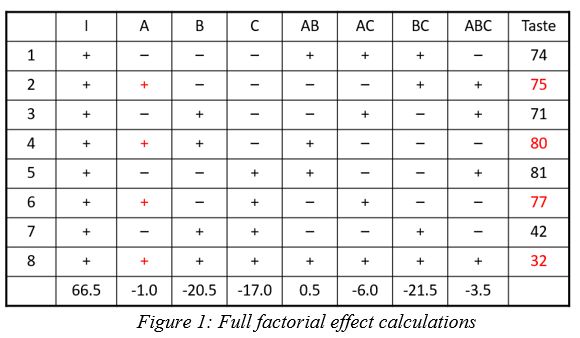Each column in the full factorial design is a unique set of pluses and minuses, resulting in independent estimates of the factor effects. An effect is calculated by averaging the response values where the factor is set high (+) and subtracting the average response from the rows where the term is set low (-). Mathematically this is written as follows: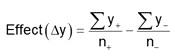In this example the A effect is calculated like this: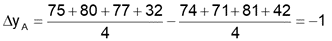The last row in figure 1 shows the calculation result for the other main effects, 2-factor and 3-factor interactions and the Identity column.

In a half-fraction design (Figure 2), only half of the runs are completed. According to standard practice, we eliminate all the runs where the ABC column has a negative sign. Now the columns are not unique – pairs of columns have the identical pattern of pluses and minuses. The effect estimates are confounded (aliased) because they are changing in exactly the same pattern. The A column is the same pattern as the BC column (A=BC). Likewise, B=AC and C=AB. Finally, I=ABC. These paired columns are said to be “aliased” with each other.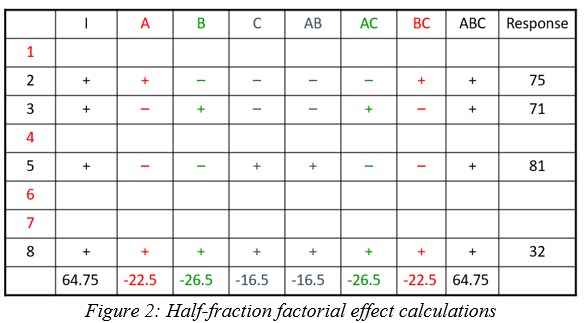In the half-fraction, the effect of A (and likewise BC) is calculated like this: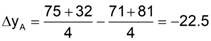When the effect calculations are done on the half-fraction, one mathematical calculation represents each pair of terms. They are no longer unique. Software may label the pair only by the first term name, but the effect is really all the real effects combined. The alias structure is written as:

I = ABC
[A] = A+BC
[B] = B+AC
[C] = C+AB

Looking back at the original data, the A effect was -1 and the BC effect was -21.5. When the design is cut in half and the aliasing formed, the new combined effect is:

A+BC = -1 + (-21.5) = -22.5

The aliased effect is the linear combination of the real effects in the system. Aliasing of main effects with two-factor interactions (2FI) is problematic because 2FI’s are fairly likely to be significant in today’s complex systems. If a 2FI is physically present in the system under study, it will bias the main effect calculation. Any system that involves temperature, for instance, is extremely likely to have interactions of other factors with temperature. Therefore, it would be critical to use a design table that has the main effect calculations separated (not aliased) from the 2FI calculations.

What type of fractional-factorial designs are “safe” to use? It depends on the purpose of the experiment. Screening designs are generally run to correctly identify significant main effects. In order to make sure that those main effects are correct (not biased by hidden 2FI’s), the aliasing of the main effects must be with three-factor interactions (3FI) or greater. The alias structure looks something like this (only main effect aliasing shown):

I = ABCD
[A] = A+BCD
[B] = B+ACD
[C] = C+ABD
[D] = D+ABC

If the experimental goal is characterization or optimization, then the aliasing pattern should ensure that both main effects and 2FI’s can be estimated well. These terms should not be aliased with other 2FI’s.

Within Design-Expert or Stat-Ease 360 software, color-coding on the factorial design selection screen provides a visual signal. Here is a guide to the colors, listed from most information to least information:

• White squares – full factorial designs (no aliasing)
• Green squares – good estimates of both main effects and 2FI’s
• Yellow squares – good estimates of main effects, unbiased from 2FI’s in the system
• Red squares – all main effects are biased by any existing 2FI’s (not a good design to properly identify effects, but acceptable to use for process validation where it is assumed there are no effects).

Good luck with your DOE data analysis!

## Christmas Trees on my Effects Plot?

posted by Shari Kraber on Dec. 3, 2020

As a Stat-Ease statistical consultant, I am often asked, “What are the green triangles (Christmas trees) on my half-normal plot of effects?”

Factorial design analysis utilizes a half-normal probability plot to identify the largest effects to model, leaving the remaining small effects to provide an error estimate. Green triangles appear when you have included replicates in the design, often at the center point. Unlike the orange and blue squares, which are factor effect estimates, the green triangles are noise effect estimates, or “pure error”. The green triangles represent the amount of variation in the replicates, with the number of triangles corresponding to the degrees of freedom (df) from the replicates. For example, five center points would have four df, hence four triangles appear. The triangles are positioned within the factor effects to reflect the relative size of the noise effect. Ideally, the green triangles will land in the lower left corner, near zero. (See Figure 1). In this position, they are combined with the smallest (insignificant) effects and help position the red line. Factor effects that jump off that line to the right are most likely significant. Consider the triangles as an extra piece of information that increases your ability to find significant effects.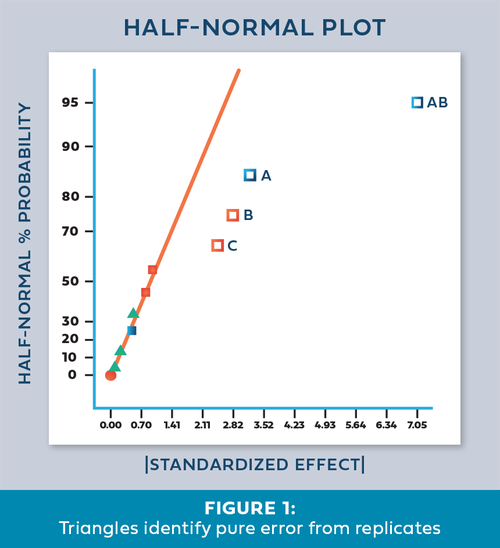Once in a while we encounter an effects plot that looks like Figure 2. “What does it mean when the green triangles are out of place - on the upper right side instead of the lower left?”

This indicates that the variation between the replicates is greater than the largest factor effects! Since this error is part of the normal process variation, you cannot say that any of the factor effects are statistically significant. At this point you should first check the replicate data to make sure it was both measured and recorded correctly. Then, carefully consider the sources of process variation to determine how the variation could be reduced. For a situation like this, either reduce the noise or increase the factor ranges. This generates larger signals that allow you to discover the significant effects.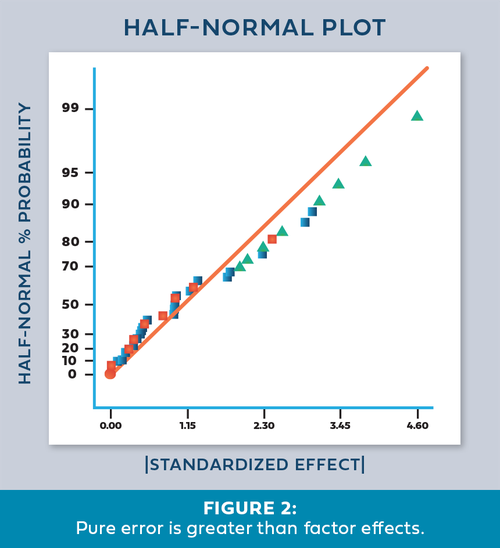- Shari Kraber

For statistical details, read “Use of Replication in Almost Unreplicated Factorials” by Larntz and Whitcomb.

## Greg’s DOE Adventure - Factorial Design, Part 1

posted by Greg on Sept. 25, 2019

[Disclaimer: I’m not a statistician. Nor do I want you to think that I am. I am a marketing guy (with a few years of biochemistry lab experience) learning the basics of statistics, design of experiments (DOE) in particular. This series of blog posts is meant to be a light-hearted chronicle of my travels in the land of DOE, not be a text book for statistics. So please, take it as it is meant to be taken. Thanks!]

So, I’ve gotten thru some of the basics (Greg's DOE Adventure: Important Statistical Concepts behind DOE and Greg’s DOE Adventure - Simple Comparisons). These are the ‘building blocks’ of design of experiments (DOE). However, I haven’t explored actual DOE. I start today with factorial design.

Factorial design (aka factorial DOE) allow you to experiment on many factors (oh, that’s where the name comes from!) at the same time. A simple version of this: 2 factors, each has two levels. [Mathematically, this is represented by 2 to the power of 2, or 22.] Example time! Cooking Spaghetti. The two factors are temperature of the water and cooking time in that water. Levels are high temperature (100 deg C) and low temperature (80 deg C); and short time in the water and long time in the water. What’s the reason for the experiment? Optimization of the process to make the best tasting (al dente) spaghetti.

We can illustrate like this: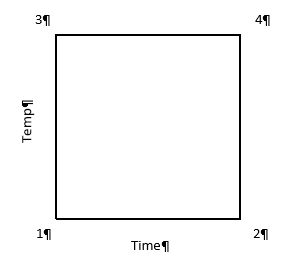In this case, the horizontal line (x-axis) is time and vertical line (y-axis) is temperature. The area in the box formed is called the Experimental Space. Each corner of this box is labeled as follows:

1 – low time, low temperature (resulting in crunchy, matchstick-like pasta), which can be coded minus-minus (-,-)

2 – high time, low temperature (+,-)

3 – low time, high temperature (-,+)

4 – high time, high temperature (making a mushy mass of nasty) (+,+)

One takeaway at this point is that when a test is run at each point above, we have 2 results for each level of each factor (i.e. 2 tests at low time, 2 tests at high time). In factorial design, the estimates of the effects (that the factors have on the results) is based on the average of these two points; increasing the statistical power of the experiment.

Power is the chance that an effect will be found, when there is an effect to be found. In statistical speak, power is the probability that an experiment correctly rejects the null hypothesis when the alternate hypothesis is true.

If we look at the same experiment from the perspective of altering just one factor at a time (OFAT), things change a bit. In OFAT, we start at point #1 (low time, low temp) just like in the Factorial model we just outlined (illustrated below).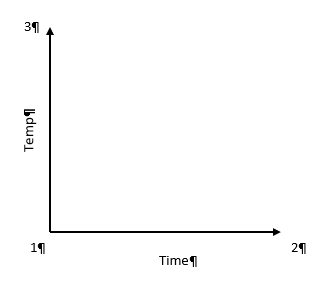Here, we go from point #1 to #2 by lengthening the time in the water. Then we would go from #1 to #3 by changing the temperature. See how this limits the number of data points we have? To get the same power as the Factorial design, the experimenter will have to make 6 different tests (2 runs at each point) in order to get the same power in the experiment.

After seeing these results of Factorial Design vs OFAT, you may be wondering why OFAT is still used. First of all, OFAT is what we are taught from a young age in most science classes. It’s easy for us, as humans, to comprehend. When multiple factors are changed at the same time, we don’t process that information too well. The advantage these days is that we live in a computerized world. A computer running software like Design-Expert®, can break it all down by doing the math for us and helping us visualize the results.

Additionally, with the factorial design, because we have results from all 4 corners of the design space, we have a good idea what is happening in the upper right-hand area of the map. This allows us to look for interactions between factors.

That is my introduction to Factorial Design. I will be looking at more of the statistical end of this method in the next post or two. I’ll try to dive in a little deeper to get a better understanding of the method.